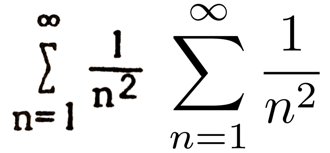Publish at April 04 2017 Updated December 16 2021

# LaTex and the basis of mathematical communication

## Symbols, syntax and mathematical terminology on the menu.Many mathematical communications assume that everyone understands the characters used, is familiar with mathematical syntax, and knows the difference between the terms used, for example, between parameters, operators, and functions.

If we once knew this, it's not clear that we still remember it. Also, the fundamental principle in mathematical communication is not to assume anything implicit in the speaker.

### Symbols

The variety of symbols used in mathematics is surprising. We start with 26 Latin and 33 Greek letters, 9 digits, four common operators (+, -, * , / ) to these are added delimiters ( ) [ ] , vectors, logical, trigonometric, matrix operators, constants (Pi, e, c, h ), characteristics (degree °, direction) and a host of other symbols, several hundred, whose meaning changes depending on the mathematical field.

In 1983, in an attempt to standardize scientific communications, Leslie Lamport proposed a standard for encoding mathematical symbols that gradually became established as software developments progressed. Today LaTex is the recognized standard. Besides making it easier to read and reproduce, LaTex is often the only format accepted by scientific publishers.

Just as it would be utopian to learn a language without knowing its alphabet, it seems equally utopian to attempt to understand math without mastering its symbols. Basically, all usable characters are in the LaTex alphabet. We can start there. ∑ ∂ ƒ˚ ...

The fact that they are not defined demonstrates the need to make their meaning clear in the context in which they are used. The meaning the author gives them may differ from the meaning the student gives them.

### Terminology

The development of mathematical jargon has accelerated over the last century. New concepts of space and dimensions have appeared in addition to imaginary numbers and complex operators. Some of these concepts are open to interpretation; therefore, it becomes essential to go back to their original definition in order to use them correctly.

Here again, to assume that the student has mastered them and has the same definition as the teacher is to take a risk of losing it along the way. For example, you are assumed to have learned about sets of numbers. What are the differences between natural, relative, rational, real, complex, imaginary numbers? If I provide a reminder, we are likely to understand each other better.

### Syntax

Syntax defines the relationships between the elements of an equation. ( 3 * 4 ) + 12 is not the same as 3 * ( 4 + 12 ) . 3 * 4 + 12 is therefore an ambiguous expression unless you define the order of precedence of the operators. This is the case with almost all operators: their order is important most of the time. Statistics, sets, logic, differential calculus each have their own syntax.

Here too, a syntactic reminder is an essential part of understanding. Not everyone remembers precisely the syntactic principles used.

Explicitly providing the elements for understanding the language makes for a successful mathematical explanation. The exercise is arduous, but profitable.

References

LaTex - Wikipedia - https://fr.wikipedia.org/wiki/LaTeX

LaTex forms - https://fr.wikipedia.org/wiki/Aide:Formules_TeX

Mathematical symbols - Wikipedia
https://fr.wikipedia.org/wiki/Table_de_symboles_math%C3%A9matiques

### Files

• Analogies

• The lessons
• The learning resources
• The file of the week
• The events
• The technologies